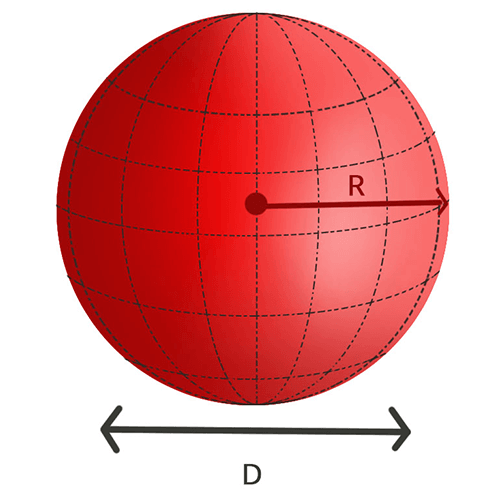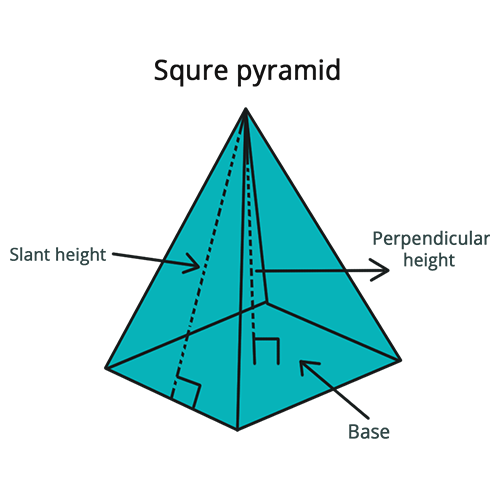• Call Now

1800-102-2727•

# Three Dimensional Shapes - Maths

Geometry is a branch of mathematics in which it deals with different shapes and sizes of different figures that can be both two-dimensional and three-dimensional. These 3D shapes are usually solid structures with a rigid or flexible body with a specific volume inside them. They have three dimensions such as length, height and breadth. There are a lot of 3D objects that can be seen in our daily life. Some of the most common ones are ice cubes, cardboard boxes, Rubik's cubes, balls, gas cylinders, cone-shaped ice cream etc.

Geometry is one of the most practical sections in maths, which involves calculating or studying various shapes and sizes and their unique properties. Geometry is generally divided into two types, one is plane geometry, and the other one is solid geometry. Plane geometry involves two-dimensional shapes and figures, namely curves, polygons, lines, circles etc. which can be drawn on paper. Meanwhile, solid geometry is the one that contains 3-dimensional objects and their properties.

Usually, three-dimensional figures have different attributes like faces, edges, vertices etc. For example, the face is nothing but a flat surface. The line segment in which the two faces meet is called an edge, whereas a vertex is a point where three edges of the figure meet.

Following are the basic three-dimensional figures:

## 1. Cube

A cube is nothing but a 3-dimensional object that contains six faces. All the faces are square-shapes.### Features of Cube

The below mentioned are the properties of a cube.

• Six faces
• Eight vertices
• 12 edges (all are equal)

## 2. Cuboid

A cuboid contains rectangular faces, unlike a cube. This can also be termed a rectangular prism. All the angles present in a cuboid measures 90°.### Features of a Cuboid

The list below has the most common properties of a cuboid.

• Six faces
• Eight vertices
• 12 edges

## 3. Cone

A cone is a solid object which contains a circular base and only has a single vertex. The figure cone can decrease smoothly from one circular flat base to the topmost point, termed the apex.### Features of a Cone

These are the properties a cone can possess.

• One circular flat face
• One edge
• One vertex
• One curved face

## 4. Cylinder

A cylinder is formed by connecting two circular flat bases to a curved body.### Features of Cylinder

These are all the metrics a cylinder contains.

• One curved face
• No vertex
• Two edges
• Two circular flat faces

## 5. Sphere

A sphere is a very common 3D solid figure perfectly round in shape and has all points on its surface as equidistant from the point considered the centre. Thus, the radius can be measured from any point on the circumference to the sphere's centre.### Features of a Sphere

The sphere contains the qualities mentioned below.

• No edges
• No vertex
• One face – curved

## 6. Pyramid

A pyramid is a basic solid structure with triangular outer faces and meets at a single point on the top. The base of a pyramid can be of any shape, namely, circular, triangular etc. For Eg, a square-based pyramid has,### Features of a Square-based Pyramid

The below mentioned are the properties of a square-based pyramid.

• Five faces
• Eight edges
• Five verticesTalk to our expert
Resend OTP Timer =
By submitting up, I agree to receive all the Whatsapp communication on my registered number and Aakash terms and conditions and privacy policy